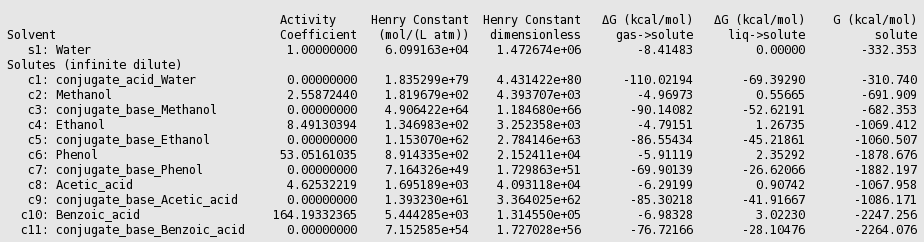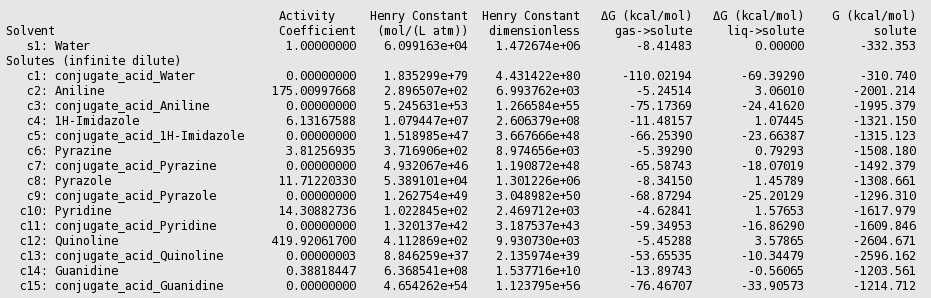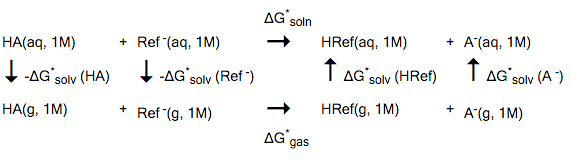# pKa values¶

In the literature one can find several strategies to calculate pKa values. Some of these strategies involve the inclusion of explicit solvent molecules, in combination with a continuum model, like COSMO. The strategies described in the next examples do not take into account explicit solvent molecules, only the continuum model is included.

## 5.1: Empirical pKa calculation method¶

In Refs. [511,512] one can find several strategies to calculate pKa values. The strategy described in this example does not take into account explicit solvent molecules, only the continuum model is included. Like in Ref.  an empirical fit is used to correlate calculated values with experimental pKa values, to account for systematic errors. The largest errors are probably present in the calculation of the solvation energies of the charged species. In these cases one probably should include explicit solvent molecules, however, that may introduce other sources of errors, and this will not be investigated here. The calculation of pKa values of acids (HA) and bases (B) is based on the following reaction model:

(acids) HA (aq, 1M) + H2O (l, 1M) → H3O+ (aq, 1M) + A- (aq, 1M) (5.1.1)
(bases) HB+ (aq, 1M) + H2O (l, 1M) → H3O+ (aq, 1M) + B (aq, 1M) (5.1.2)

The free energy of dissociation for acids and bases is calculated as

(acids) $$\Delta$$ G*diss = G(A-) - G(HA) + G(H3O+) - G(H2O) (5.1.3)
(bases) $$\Delta$$ G*diss = G(B) - G(HB+) + G(H3O+) - G(H2O) (5.1.4)

The * denotes a standard state of 1M (1 mol/L). The pKa can then be calculated as (see for example Refs. [511,512])

pKa = $$\Delta$$ G*diss/(RT ln(10)) - 1.74 (5.1.5)

At T = 298.15, 1/(RT ln(10)) = 0.733 mol/kcal. The term -1.74 is to correct for the standard state of liquid water, which is 55 mol/L.

Emperical fit

Like in Ref.  instead of this equation (5.1.5), a linear fit has been made by correlating the calculated $$\Delta$$ G* diss values with experimental pKa values, to account for systematic errors that are present in this method. For acids and bases a different empirical adjusted equation will be used, optimized for the ADF COSMO-RS implementation:

(acids) pKa = 0.62 $$\Delta$$ G*diss/(RT ln(10)) + 2.10 (5.1.6)
(bases) pKa = 0.67 $$\Delta$$ G*diss/(RT ln(10)) - 2.00 (5.1.7)

These fitted parameters are not so far from the fitted parameters in Ref. . Zero-point vibrational energies have not been taken into account in the calculation of the free energy of dissociation. H3 O+ (Hydronium ion) is the conjugate acid of Water. Molecules can have two or more equivalent sites for protonation or deprotonation are also not taken into account, which can have an effect on the pKa value. However, such effects are not taken into account here. Like for neutral compounds, one should optimize the anions and cations in the gas phase, and use this geometry also in the COSMO calculation. It is important to choose the lowest energy conformer. In the example below the molecules have a single relevant conformation for the protonated and deprotonated form.

Acids

Copy the .coskf files which are listed in $AMSHOME/examples/COSMO-RS/pKa/tutorial5.1_acid.compoundlist and the file tutorial5.1_acid.compoundlist to the directory Tutorial. The tutorial5.1_acid.compoundlist is a file with a list of acids and their conjugate bases that is limited to the compounds needed in this example. In these .coskf files already the correct number of ring atoms is included. Open a new COSMO-RS GUI window Add the compounds listed in tutorial5.1_acid.compoundlist Select Properties → Activity coefficients Select ‘Water’ for the first component in Solvent Enter ‘298.15’ for ‘Temperature Kelvin’ Check the ‘+’ button to add the first 12 compounds from the list Press ‘Run’Using equation 5.1.6 (pKa = 0.62*0.733* $$\Delta$$ Gdiss +2.10), with $$\Delta$$ Gdiss = G(conjugate_base_acid) - G(acid) + G(conjugate_acid_Water) - G(Water) in kcal/mol, results can be put in a table. Acid experimental pK a  calculated pK a 1 Methanol 15.5 16.27 2 Ethanol 15.9 15.97 3 Phenol 9.82 10.32 4 Acetic acid 4.75 3.64 5 Benzoic acid 4.27 4.28 Bases Copy the .coskf files which are listed in$AMSHOME/examples/crs/Tutorial5/tutorial5.1_base.compoundlist and the file tutorial5.1_base.compoundlist the directory Tutorial. The tutorial5.1_base.compoundlist is a file with a list of bases and their conjugate acids that is limited to the compounds needed in this example. In these .coskf files already the correct number of ring atoms is included.

Open a new COSMO-RS GUI window
Add the compounds listed in tutorial5.1_base.compoundlist
Select Properties → Activity coefficients
Select ‘Water’ for the first component in Solvent
Enter ‘298.15’ for ‘Temperature Kelvin’
Check the ‘+’ button to add the first 16 compounds from the list
Press ‘Run’Using equation 5.1.7 ((pKa = 0.67*0.733* $$\Delta$$ Gdiss -2.00), with $$\Delta$$ Gdiss = G(base) - G(conjugate_acid_base) + G(conjugate_acid_Water) - G(Water) in kcal/mol, results can be put in a table.

Base experimental pK a  calculated pK a
1 Aniline 4.6 5.75
2 1H-Imidazole 7 5.65
3 Pyrazine 0.7 0.85
4 Pyrazole 2.5 2.55
5 Pyridine 5.14 4.62
6 Quinoline 4.80 4.43
7 Guanidine 13.8 14.09
1. J. Ho and M.L. Coote, A universal approach for continuum solvent pKa calculations: are we there yet?, Theoretical Chemistry Accounts 125, 3 (2010)
1. F. Eckert, M. Diedenhofen, and A. Klamt, Towards a first principles prediction of pKa : COSMO-RS and the cluster-continuum approach, Molecular Physics 108, 229 (2010)

## 5.2: Relative pKa calculation method¶

The method described in this example is based on one of the strategies in Ref. . This method uses the experimental pKa value of a reference compound, experimental gas phase deprotonation energies, and COSMO-RS solvation free energies. A suitable reference compound (HRef) should be chosen, which is similar to the actual compound (HA) one is interested in. For example, the deprotonation reaction could be similar in both compounds. The calculation of pKa values is based on the following reaction model:The * denotes a standard state of 1M (1 mol/L). The free energy of solvation is then calculated as

$$\Delta$$ G* soln = $$\Delta$$ G* gas + $$\Delta$$ G* solv (HRef) + $$\Delta$$ G* solv (A- ) - $$\Delta$$ G* solv (HA) - $$\Delta$$ G* solv (Ref - )

The pKa can then be calculated as (see for example Ref. )

pKa = $$\Delta$$ G* soln /(RT ln(10)) + pKa (HRef)

The success of this method relies on the availability of a suitable reference compound with an accurately known experimental pKa value. Instead of experimental gas phase deprotonation energies one might calculate the gas phase reaction free energy $$\Delta$$ G* gas , using DFT or some high level ab initio method.

Example Ethanol

In this example the pKa value of Ethanol will be calculated. As reference compound Methanol is chosen, which has an experimental pKa value of 15.5. Experimental deprotonation energies are taken from Ref. [521, supporting information], for Methanol this is $$\Delta$$ r G0 = 1569 kJ/mol, and for Ethanol $$\Delta$$ r G0 = 1555 kJ/mol, see also, for example, Ref. . The COSMO-RS solvation free energies of Methanol, Methoxide (the conjugate base of Methanol), Ethanol, and Ethoxide (the conjugate base of Ethanol) have already been calculated in the previous tutorial 5.1. At T = 298.15, 1/(RT ln(10)) = 0.733 mol/kcal. The free energy of solvation (kcal/mol) and the pKa of Ethanol are then calculated as

$$\Delta$$ G* soln = (1555-1569)/4.184 - 4.97 - 86.56 + 4.79 + 90.14 = 0.05 kcal/mol
pKa (Ethanol) = 0.733*0.05 + 15.5 $$\approx$$ 15.5

The calculated pKa of Ethanol of 15.5 is close to the experimental value of 15.9.

1. J. Ho and M.L. Coote, A universal approach for continuum solvent pKa calculations: are we there yet?, Theoretical Chemistry Accounts 125, 3 (2010)